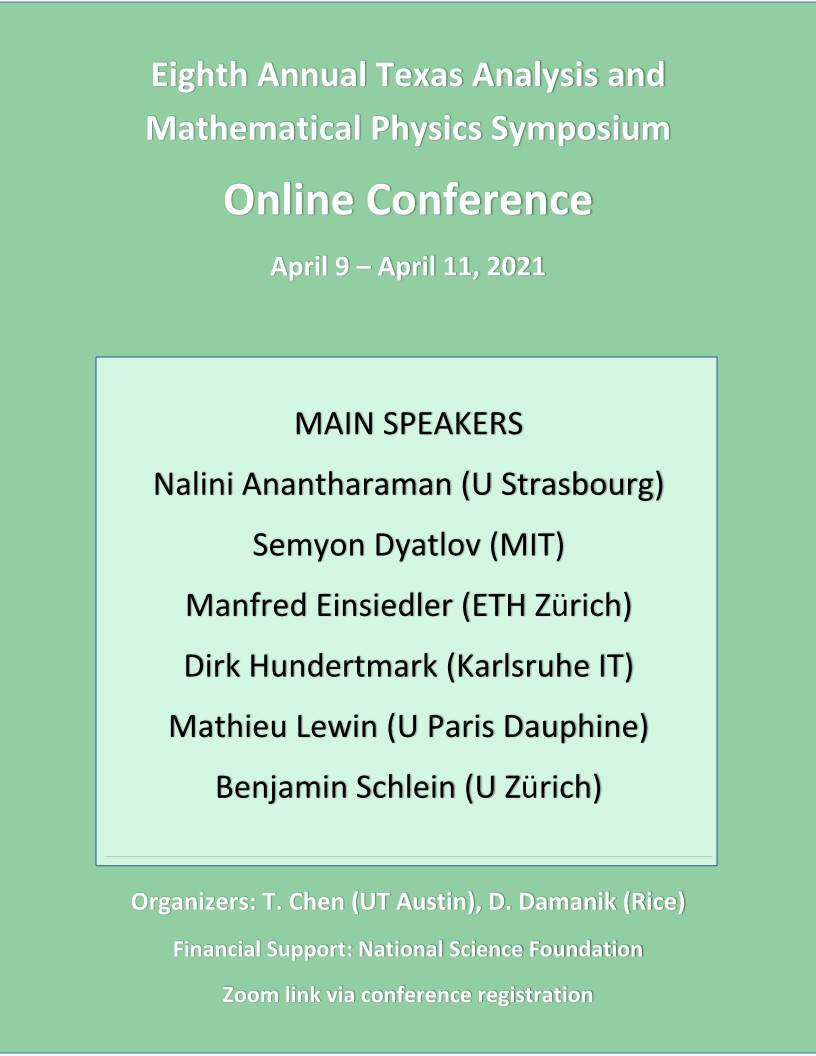# ONLINE CONFERENCE

## April 9 - April 11, 2021### Abstracts

 Nalini Anantharaman (Universite de Strasbourg) The bottom of the spectrum of a random hyperbolic surface (work in progress) In an ongoing project with Laura Monk, we are trying to prove that on a random hyperbolic surface, with high probability (and in the limit when the volume goes to infinity) there are no small eigenvalues. Most of the talk will be dedicated to giving motivations, constructing the probabilistic model, and describing the strategy to solve a similar question for random regular graphs (due to Friedman and Bordenave). Semyon Dyatlov (MIT) Control of eigenfunctions on negatively curved surfaces Given an $L^2$-normalized eigenfunction with eigenvalue $\lambda^2$ on a compact Riemannian manifold $(M,g)$ and a nonempty open set subset $\Omega$ of $M$, what lower bound can we prove on the $L^2$-mass of the eigenfunction on $\Omega$? The unique continuation principle gives a bound for any $\Omega$ which is exponentially small as $\lambda$ goes to infinity. On the other hand, microlocal analysis gives a $\lambda$-independent lower bound if $\Omega$ is large enough, i.e. it satisfies the geometric control condition. This talk presents a $\lambda$-independent lower bound for any set $\Omega$ in the case when $M$ is a negatively curved surface, or more generally a surface with Anosov geodesic flow. The proof uses microlocal analysis, the chaotic behavior of the geodesic flow, and a new ingredient from harmonic analysis called the Fractal Uncertainty Principle. Applications include control for Schrodinger equation and exponential decay of damped waves. Joint work with Jean Bourgain, Long Jin, and St\'ephane Nonnenmacher. Manfred Einsiedler (ETH Zurich) Measure Rigidity for Diagonal Actions We will survey a number of results obtained in a series of papers with Elon Lindenstrauss. These concern partial results towards conjectures raised by Furstenberg, Margulis, and Katok-Spatzier concerning classifications of invariant measures for higher rank diagonalisable actions. Dirk Hundertmark (Karlsruhe Institute of Technology) Quantum Systems at The Brink: Helium What happens if a parameter of a quantum system is tuned in such a way that a bound state energy (e.g., the ground state energy) hits the bottom of the essential spectrum? Does the eigenvalue survive, i.e., the corresponding eigenfunction stays $L^2$, or does it dissolve into the continuum energies? It turns out that a) the answer depends on the dimension, b) a long range repulsive part of the which classically should push the system apart, will stabilize the quantum system (This might sound weird, but I'll explain it :-)), and c) in particular, a long-range repulsive part of the potential helps to get WKB type decay estimates for bound states whose eigenvalues are at the bottom of the essential spectrum, when the usual approach due to Agmon does not apply. I explain the main ideas on how one can get upper bounds on the decay of eigenfunction in a one particle toy model and the case of helium at critical coupling. In both cases the well-known Agmon method does not work. Moreover, for helium at critical coupling we also derive non-isotropic upper and matching lower bounds on the asymptotic decay of the ground state at the threshold. In particular, our bounds are sharp (modulo lower order terms). The method apply to a variety of critical quantum systems, where an eigenvalue enters the continuum, but stays an eigenvalue. This is joint work with Markus Lange (UBC) and Michal Jex (Prague). Mathieu Lewin (CEREMADE, Universite Paris Dauphine) Derivation of nonlinear Gibbs measures from quantum mechanics Nonlinear Gibbs measures are probability measures in infinite dimensions, which play an important role in (S)PDE, in Quantum Field Theory and for Bose-Einstein condensates. In dimensions 2 and 3, these measures concentrate on distribution spaces, and the nonlinear term, typically of Hartree of NLS type, has to be renormalized. I will present recent results in collaboration with Phan Thanh Nam (Munich) and Nicolas Rougerie (Lyon) about the derivation of these measures from many-body quantum mechanics in a mean-field type limit. Benjamin Schlein (University of Zurich) Correlation energy of weakly interacting fermions We consider a trapped Fermi gas in the mean-field regime. Hartree-Fock theory, based on the restriction to Slater determinants, provide a first approximation for the ground state energy. In this talk, we prove the validity of a formula, predicted by Gell-Mann and Brueckner in the physics literature, for the correction to the Hartree-Fock energy resulting from the correlations among particles. Our derivation is based on an application of rigorous (bosonic) Bogoliubov theory. This talk is based on joint works with N. Benedikter, P.T. Nam, M. Porta and R. Seiringer. Return to main page